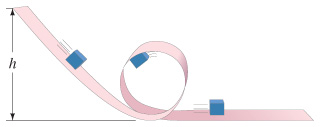# Problem: The small mass m sliding without friction along the looped track shown in the figure is to remain on the track at all times, even at the very top of the loop of radius r.If the actual release height is 4 h, calculate the normal force exerted by the track at the bottom of the loop.If the actual release height is 4 h, calculate the normal force exerted by the track at the top of the loop.If the actual release height is 6 h, calculate the normal force exerted by the track after the block exits the loop onto the flat section.Determine the minimum release height h.

⚠️Our tutors found the solution shown to be helpful for the problem you're searching for. We don't have the exact solution yet.

###### Problem Details

The small mass m sliding without friction along the looped track shown in the figureis to remain on the track at all times, even at the very top of the loop of radius r.

If the actual release height is 4 h, calculate the normal force exerted by the track at the bottom of the loop.

If the actual release height is 4 h, calculate the normal force exerted by the track at the top of the loop.

If the actual release height is 6 h, calculate the normal force exerted by the track after the block exits the loop onto the flat section.

Determine the minimum release height h.